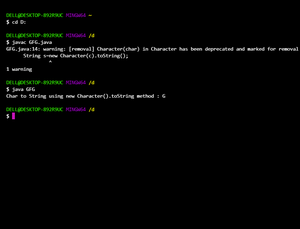Open in App
Not now

# How to Convert Char to String in Java?

• Difficulty Level : Easy
• Last Updated : 06 Dec, 2022

If we have a char value like ‘G’ and we want to convert it into an equivalent String like “G” then we can do this by using any of the following four listed methods in Java:

Illustration:

```Input  : 'G'
Output : "G"```

Methods:

There are various methods by which we can convert the required character to string with the usage of wrapper classes and methods been provided in java classes.

1. Using concatenation of strings
2. Using toString() method of Character class
3. Using Character wrapper class
4. Using valueOf() method of String class

Let us discuss these methods in detail below as follows with clean java programs as follows:

Method 1: Using concatenation of strings

We can convert a char to  a string  object in java by concatenating the given character with an empty string .

Example

## Java

 `// Java Program to Convert Char to String``// Using Concatenation in Strings` `// Importing the required packages``import` `java.io.*;``import` `java.util.*;` `// Main class``class` `GFG {` `    ``// Main driver method``    ``public` `static` `void` `main(String[] args)``    ``{` `        ``// Declaring a char variable``        ``char` `c = ``'G'``;` `        ``// Concatenating the char variable``        ``// with an empty string``        ``String s = ``""` `+ c;``      ` `        ``// Print and display the above string``        ``System.out.println(``            ``"Char to String using Concatenation :"``            ``+ ``" "` `+ s);``    ``}``}`

Output

`Char to String using Concatenation : G`

Method 2: Using toString() method of Character class

We can convert a char to a string  object in java by using the  Character.toString() method.

Example

## Java

 `// Java Program to Convert Char to String``// Using toString() method of Character class` `// Importing the required packages``import` `java.io.*;``import` `java.util.*;` `// Main class``class` `GFG {` `    ``// Main driver method``    ``public` `static` `void` `main(String[] args)``    ``{` `        ``// Declaring a char variable``        ``char` `c = ``'G'``;` `        ``// Converting the char to String using toString``        ``// method of Character class and storing it in a``        ``// string``        ``String s = Character.toString(c);` `        ``// Print and display the above string``        ``System.out.println(``            ``"Char to String using Character.toString method :"``            ``+ ``" "` `+ s);``    ``}``}`

Output

`Char to String using Character.toString method : G`

Method 3: Using Character wrapper class

We can convert a char to  a string  object in java  by using java.lang.Character class, which is a wrapper for char primitive type.

Note: This method may arise a warning due to the new keyword as Character(char) in Character has been deprecated and marked for removal.

Example

## Java

 `// Java Program to Convert Char to String``// Using toString() method of Character class` `// Importing the required packages``import` `java.io.*;``import` `java.util.*;` `// Main class``class` `GFG {` `    ``// Main driver method``    ``public` `static` `void` `main(String[] args)``    ``{` `        ``// Declaring a char variable``        ``char` `c = ``'G'``;` `        ``// Converting the char to String using toString``        ``// method of Character class and storing it in a``        ``// string``        ``String s = Character.toString(c);` `        ``// Print and display the above string``        ``System.out.println(``            ``"Char to String using Character.toString method :"``            ``+ ``" "` `+ s);``    ``}``}`Method 3-A

Using @deprecated annotation with Character.toString(). This will help you to avoid warnings and let you use deprecated methods . This will help you remove the warnings as mentioned in Method 3

## Java

 `// Importing the required packages``import` `java.io.*;``import` `java.util.*;` `// Main class``class` `GFG {` `    ` `  ``@Deprecated``    ``// Main driver method``    ``public` `static` `void` `main(String[] args)``    ``{` `        ``Character ch=``new` `Character(``'G'``);` `        ``// Print and display the above string``        ``System.out.println(``            ``"Char to String using @Deprecated Annotation and toString method :"``            ``+ ``" "` `+  ch.toString());``      ` `    ``/*  This method will arise a warning since the new keyword in Character(char)``        ``in Character has been deprecated and marked for removal. That is why we``        ``have used @Deprecated annotation``      ``*/``    ``}``}`

Output

`Char to String using @Deprecated Annotation and toString method : G`

Method 4-A: Using String.valueOf() method of String class

We can convert a char to  a string  object in java  by using String.valueOf(char[]) method.

Example

## Java

 `// Java Program to Convert Char to String``// Using String.valueOf() method of String class` `// Importing the required packages``import` `java.io.*;``import` `java.util.*;` `// Main class``class` `GFG {` `    ``// Main driver method``    ``public` `static` `void` `main(String[] args)``    ``{` `        ``// Declaring a char variable``        ``char` `c = ``'G'``;` `        ``// Converting char to String by``        ``// using String.valueOf() method``        ``String s = String.valueOf(``new` `char``[] { c });` `        ``// Print and display the above-stored string``        ``System.out.println(``            ``"Char to String using String.valueOf(new char[]) method :"``            ``+ ``" "` `+ s);``    ``}``}`

Output

`Char to String using String.valueOf(new char[]) method : G`

Method 4-B: Using valueOf() method of String class

We can convert a char to  a string  object in java  by using String.valueOf() method.

Example

## Java

 `// Java Program to Convert Char to String``// Using valueOf() method of String class` `// Importing the required packages``import` `java.io.*;``import` `java.util.*;` `// Main class``class` `GFG {` `    ``// Main driver method``    ``public` `static` `void` `main(String[] args)``    ``{``        ``// Declaring a char variable``        ``char` `c = ``'G'``;``      ` `        ``// Converting char to String``        ``// using String.valueOf() method``        ``String s = String.valueOf(c);` `        ``// Print and display the String s``        ``System.out.println(``            ``"Char to String using String.valueOf() method :"``            ``+ ``" "` `+ s);``    ``}``}`

Output

`Char to String using String.valueOf() method : G`

My Personal Notes arrow_drop_up